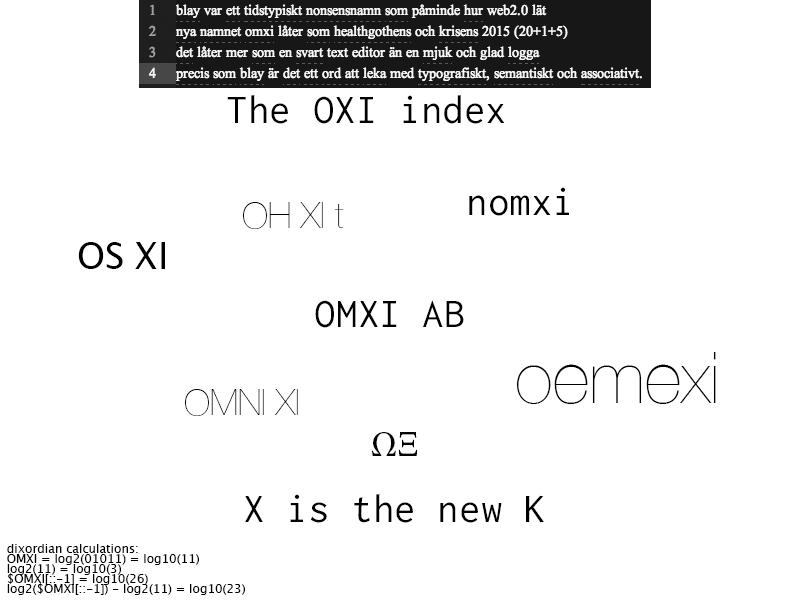# Blay##### philoxiphyDu som läser det här har insett att bloggen har bytt namn från blay.se till omxi.se. Blay var ett tidstypiskt nonsensnamn som påminde om hur web2.0 lät.
Nya namnet omxi låter som healthgothens och krisen 2015 (20+1+5)
det låter mer som en svart text editor än en mjuk och glad logga
precis som blay är det ett ord att leka med typografiskt, semantiskt, och associativt.

If you are reading this, you realize that the blog has changed name from blay.se to omxi.se. Blay was a timely nonsense name that that reminded of how web2.0 sounded. The new name ‘omxi.se’ sounds more like the healthgoth and crisis of 2015 (20+1+5). It sounds more like a black text editor than a soft and friendly logo. Just like blay, it is a word to play with typographically, semantically, and associatively.

# ΩΞ

OMXI can also be written as the greek letters Omega (Ω) and Xi (Ξ), none of which have latin equivalents.

Ω is the last letter in the Greek alphabet. Ξ resides between N and O. The space in NO (OXI in Greek).

Ω as the broken circle, o meets m, resistance to circulation, friction, the ultimate limit of a set, the end of everything, memento omxi.

Ξ as in:

• The “cascade particles” in particle physics
• Indicating “no change of state” in Z notation
The partition function under the grand canonical ensemble in statistical - mechanics
• Harish-Chandra’s Ξ function
• The Riemann Xi function
• Ether currency of Ethereum
• Random variables
• Dimensionless distance variable used in the Lane–Emden equation
Extent of reaction, a concept in physical chemistry used most often in - chemical engineering kinetics
• Eigenvectors
• Damping Ratio C/Ccr(vibrational analysis)
• A parameter in a generalized Pareto distribution
The symmetric function equation of the Riemann zeta function in mathematics, - also known as the Riemann Xi function
• A universal set in set theory
A number used in the remainder term of Taylor’s theorem that falls between - the limits a and b
A number used in error approximations for formulas that are applications of - Taylor’s theorem, such as Newton–Cotes formulas
One of the two different polypeptide chains of the human embryonic hemoglobin - types Hb-Portland (ξ2γ2) and Hb-Gower I (ξ2ε2)
• The correlation function in astronomy
• Spatial frequency; also sometimes temporal frequency.
• A small displacement in MHD plasma stability theory
A parameter denoted as warped time used to derive the equations for - homogeneous azeotropic distillation
The x-coordinate of computational space as used in computational fluid - dynamics
• Potential difference in physics (in volts)
• The radial integral in the spin-orbit matrix operator in atomic physics.
• The Killing vector in general relativity.
Average logarithmic energy decrement per collision (neutron calculations in - nuclear physics)
• Pippard’s cohesion length in superconductors
• State Price Density in mathematical finance
• The diameter of a crystal nucleus in nucleation theory

# On the Omni Cross Interflexers (OXI)

The Omni Cross Interflexers (OXI), formerly knows as the World Wide Web (WWW)

The Internet Ω Ξ, formerly knows as InternetZero

@omxi on twitter is an egg called kLaUdItHa XiOmArA

OMXI is the Icelandic branch of the NASDAQ OMX Group

OMXI = log2(01011) = log10(11)
log2(11) = log10(3)
\$OMXI[::-1] = log10(26)
log2(\$OMXI[::-1]) - log2(11) = log10(23)